Question

# Q.4 A position control system is shown in Figure Q4. Assume that K(s) = K, the plant 50 s(0.2s +1) transfer function is given by G(s) s02s y(t) r(t) Figure Q4: Feedback control system. (a) Design a l...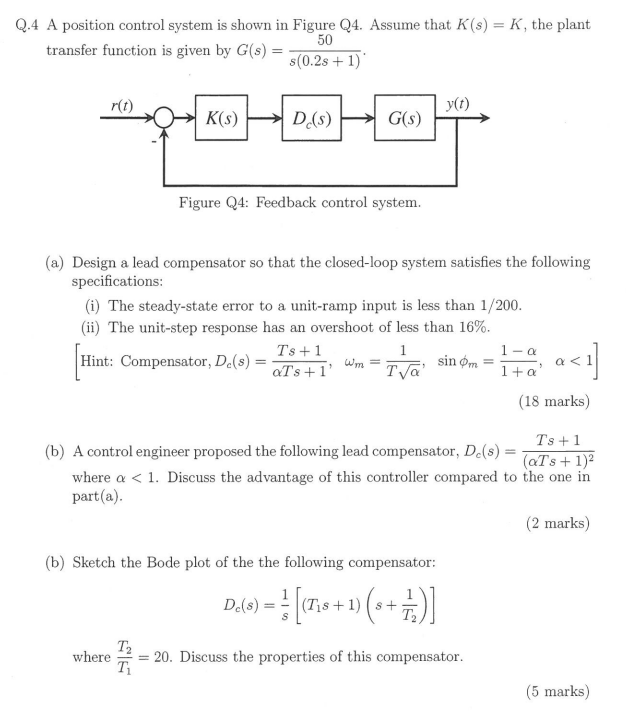Q.4 A position control system is shown in Figure Q4. Assume that K(s) = K, the plant 50 s(0.2s +1) transfer function is given by G(s) s02s y(t) r(t) Figure Q4: Feedback control system. (a) Design a lead compensator so that the closed-loop system satisfies the following specifications (i) The steady-state error to a unit-ramp input is less than 1/200 (ii) The unit-step response has an overshoot of less than 16% Ts +1 Hint: Compensator, Dc(s)=aTs+ 1, wm-T (18 marks) Ts 1 aTs 1)2 (b) A control engineer proposed the following lead compensator, De(s) where α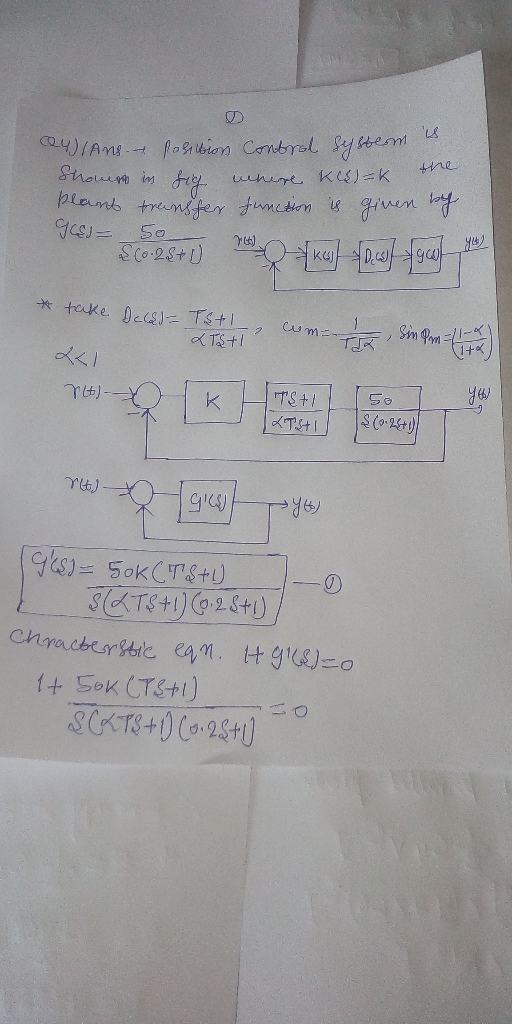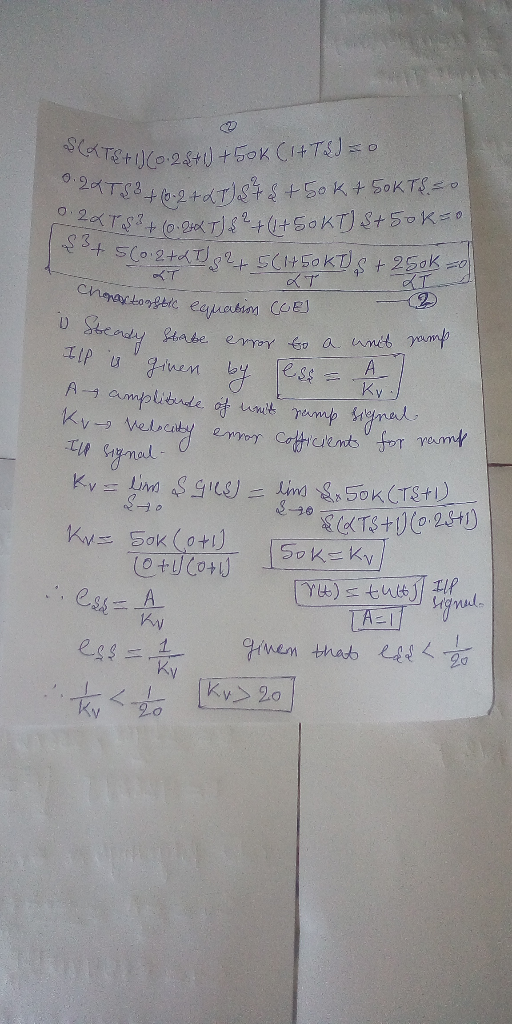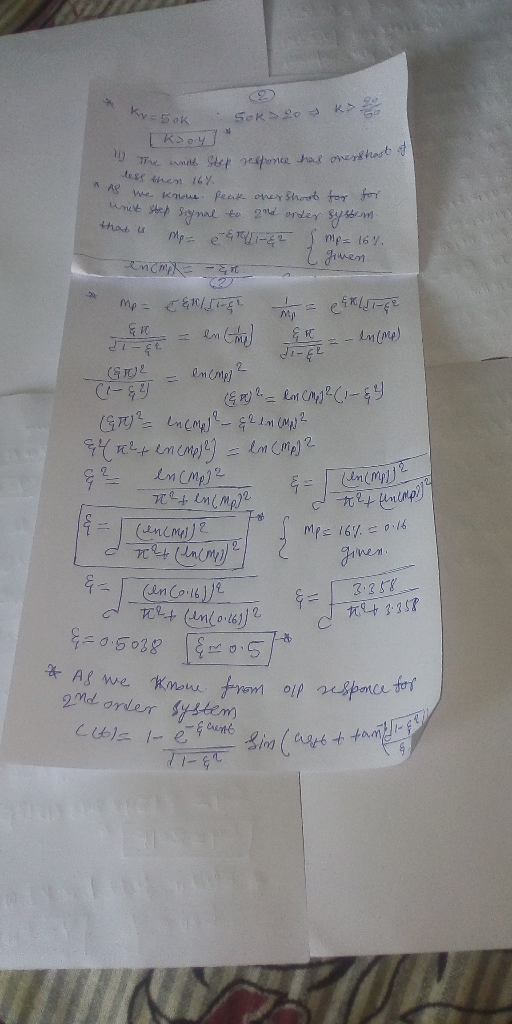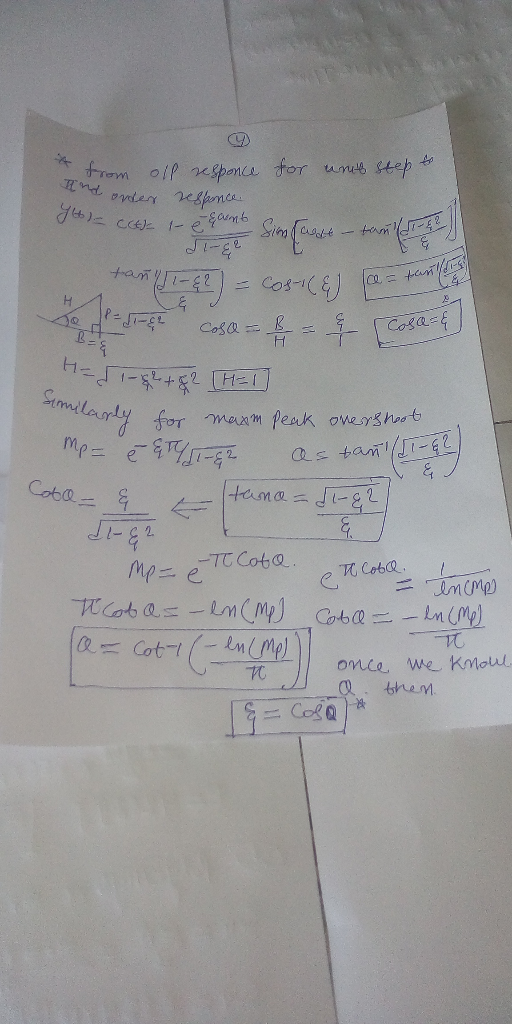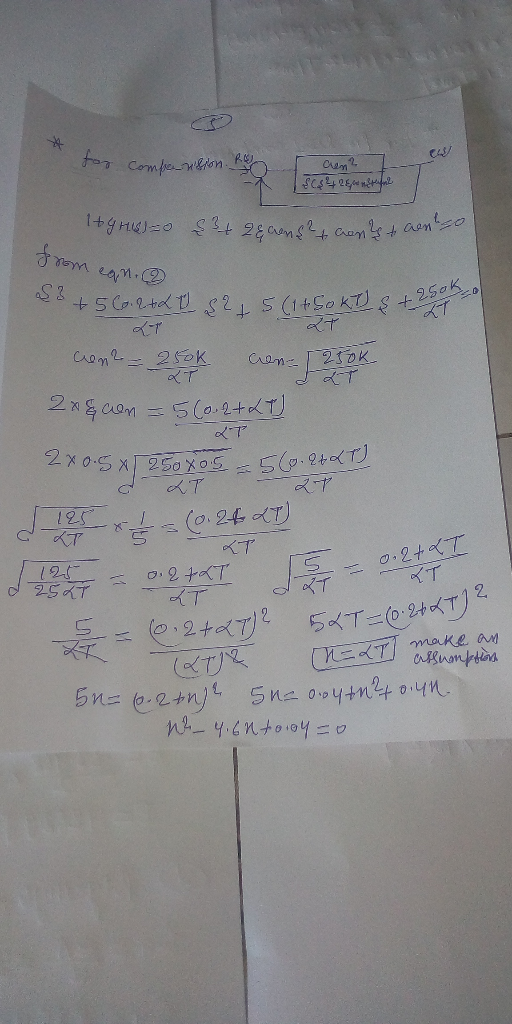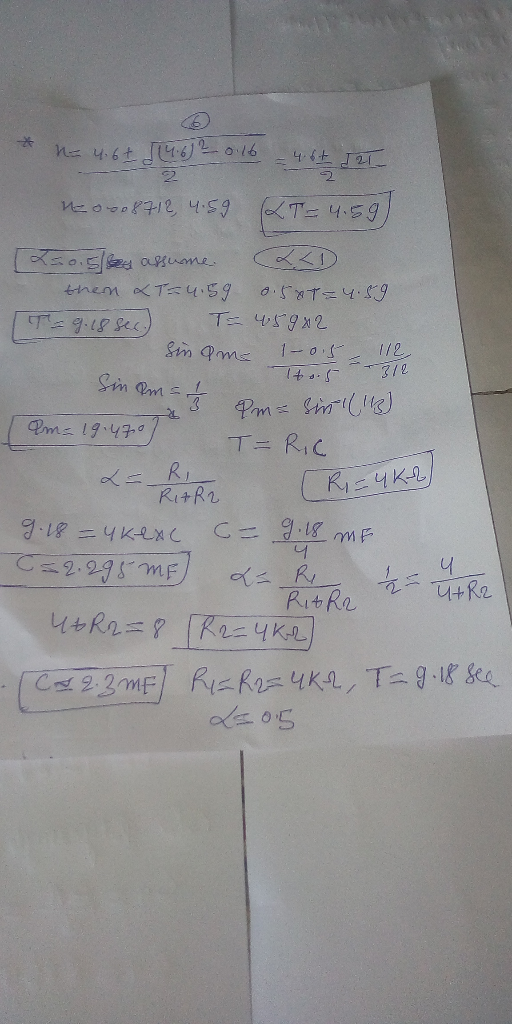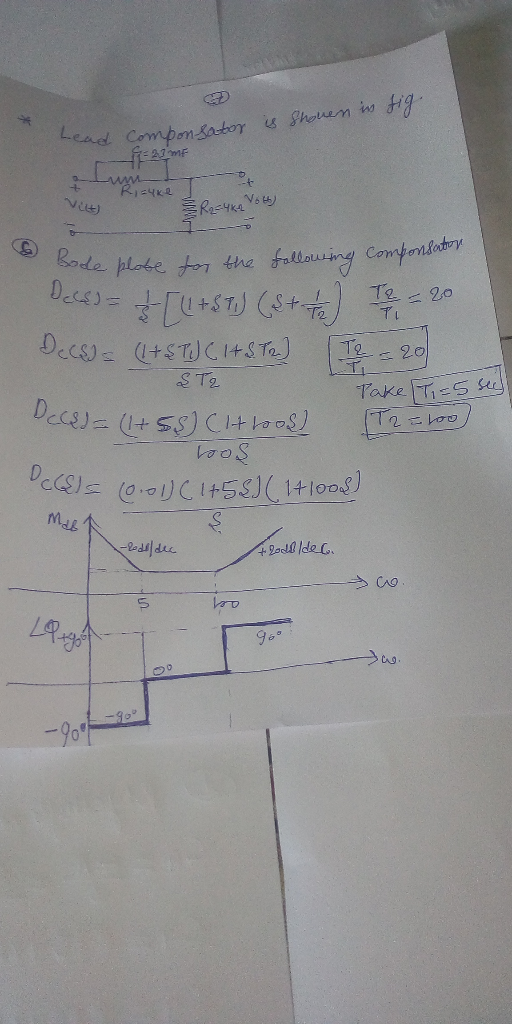#### Earn Coins

Coins can be redeemed for fabulous gifts.

Similar Homework Help Questions
• ### Consider the transfer function of a DC motor given by G(s) = 1 / s(s+2) 3. Consider the transfer function of a DC motor...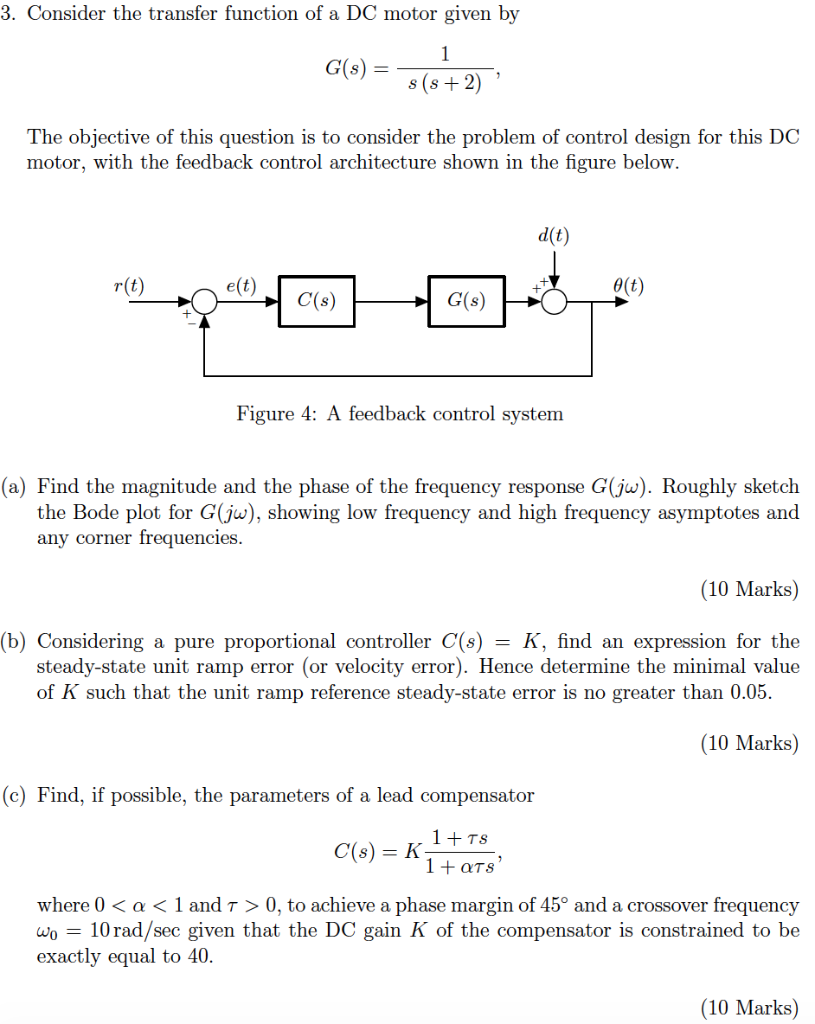Consider the transfer function of a DC motor given by G(s) = 1 / s(s+2) 3. Consider the transfer function of a DC motor given by 1 G(s) s (s2) The objective of this question is to consider the problem of control design for this DC motor, with the feedback control architecture shown in the figure below d(t r(t) e(t) e(t) C(s) G(s) Figure 4: A feedback control system (a) Find the magnitude and the phase of the frequency response...

• ### Problem 4. The open-loop transfer function of a unity feedback system is 20 G(s) S+1.5) (s +3.5) ...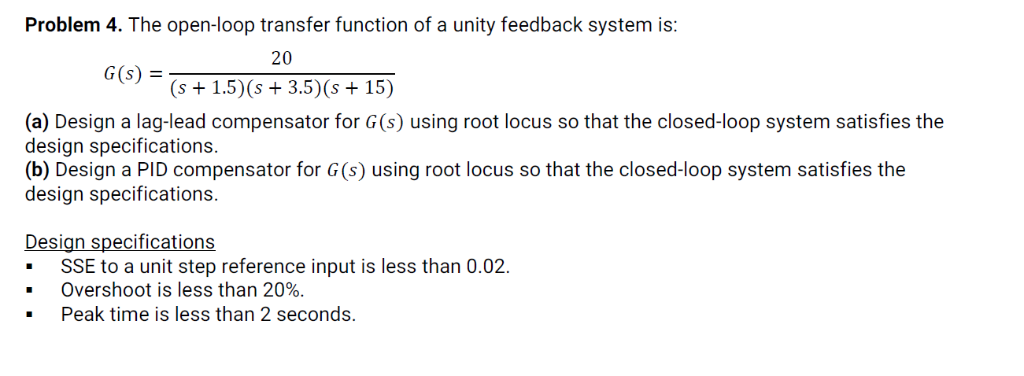Problem 4. The open-loop transfer function of a unity feedback system is 20 G(s) S+1.5) (s +3.5) (s +15) (a) Design a lag-lead compensator for G(s) using root locus so that the closed-loop system satisfies the design specifications. (b) Design a PID compensator for G(s) using root locus so that the closed-loop system satisfies the design specifications. Design specifications -SSE to a unit step reference input is less than 0.02. Overshoot is less than 20%. Peak time is less than...

• ### Problem 4. The open-loop transfer function of a unity feedback system is: 20 (s+1.5)(s 3.5) (s 15...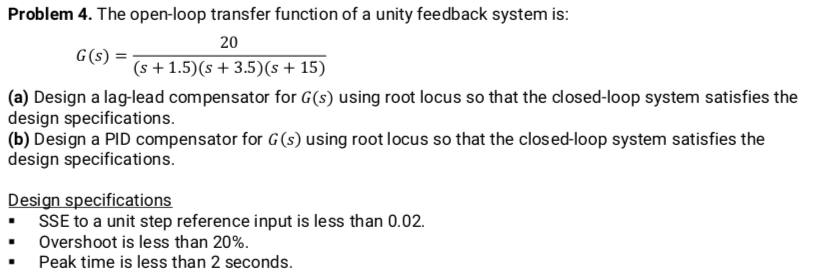Problem 4. The open-loop transfer function of a unity feedback system is: 20 (s+1.5)(s 3.5) (s 15) G(s) (a) Design a lag-lead compensator for G(s) using root locus so that the closed-loop system satisfies the design specifications (b) Design a PID compensator for G (s) using root locus so that the clos ed-loop system satisfies the design specifications. Design specifications .SSE to a unit step reference input is less than 0.02. Overshoot is less than 20% Peak time is less...

• ### Consider a system modelled by means of the following transfer function 10 G(s) s(s +1)(s +10)...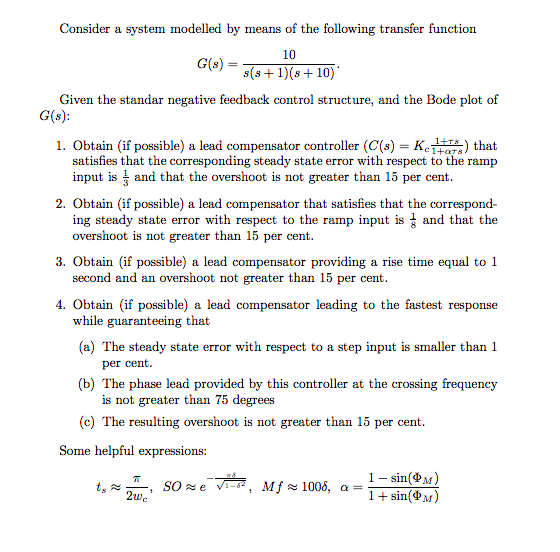Consider a system modelled by means of the following transfer function 10 G(s) s(s +1)(s +10) Given the standar negative feedback control structure, and the Bode plot of G(s): 1. Obtain (if possible) a lead compensator controller (C(s) Kc1+ts) that satisfies that the corresponding steady state error with respect to the ramp input is and that the overshoot is not greater than 15 per cent 2. Obtain (if possible) a lead compensator that satisfies that the correspond- ing steady state...

• ### Lag Compensator Design Using Root-Locus 2. Consider the unity feedback system in Figure 1 for G(s...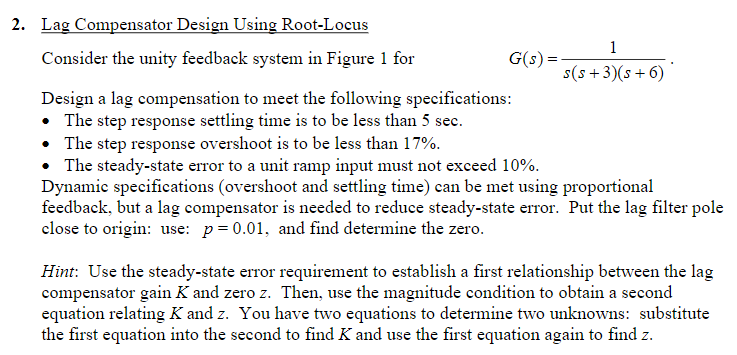Lag Compensator Design Using Root-Locus 2. Consider the unity feedback system in Figure 1 for G(s)- s(s+3(s6) Design a lag compensation to meet the following specifications The step response settling time is to be less than 5 sec. . The step response overshoot is to be less than 17% . The steady-state error to a unit ramp input must not exceed 10%. Dynamic specifications (overshoot and settling time) can be met using proportional feedback, but a lag compensator is needed...

• ### 10 Q.1 Figure Q1 shows a speed control system where Gi(s) 0.5s 1' and K(s)kp K(s) G,(s) Figure Q1: Speed Control System a) Determine the transfer function from d to y (4 marks) (b) Assuming the r...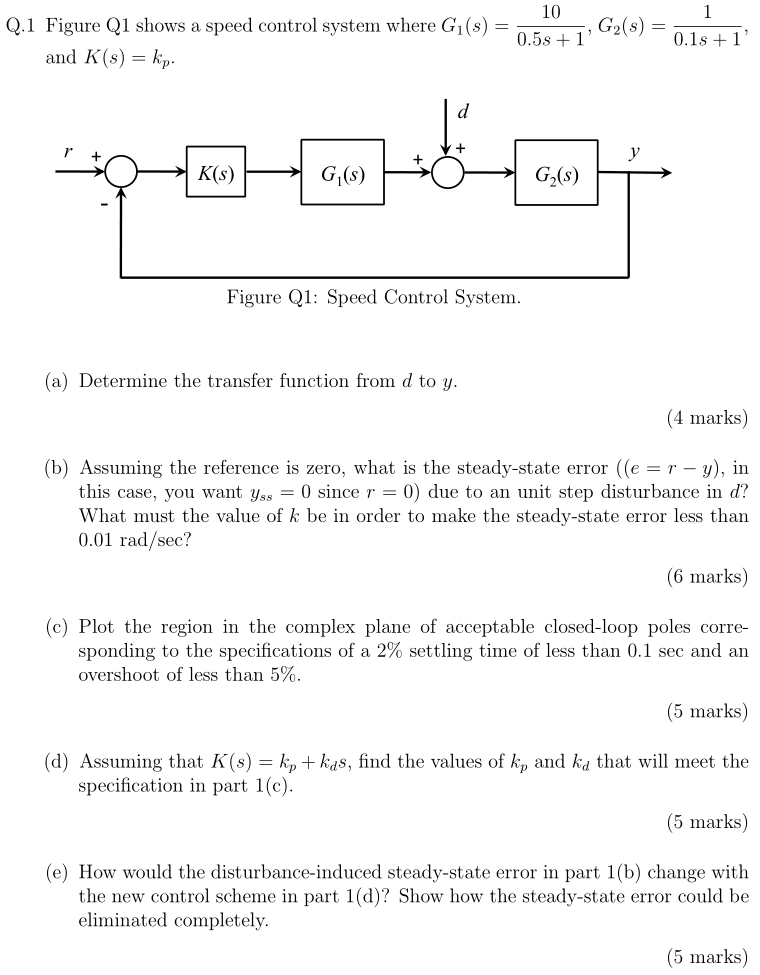10 Q.1 Figure Q1 shows a speed control system where Gi(s) 0.5s 1' and K(s)kp K(s) G,(s) Figure Q1: Speed Control System a) Determine the transfer function from d to y (4 marks) (b) Assuming the reference is zero, what is the steady-state error (e-r - y), in this case, you want yss since r 0) due to an unit step disturbance in d? What must the value of k be in order to make the steady-state error less than...

• ### urgent!! II Lag/lead Compensator Design A certain plant with unity feedback has the model given by GP(s) s(1 +0.1s) (1 0.2s) Design a phase-lag OR phase-lead compensator such that: 1. The steady-...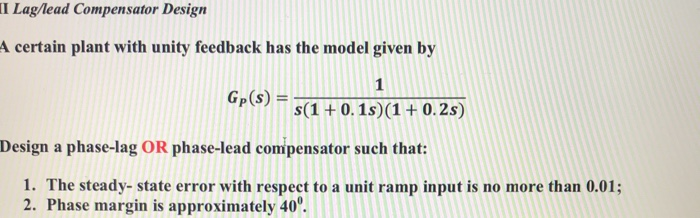urgent!! II Lag/lead Compensator Design A certain plant with unity feedback has the model given by GP(s) s(1 +0.1s) (1 0.2s) Design a phase-lag OR phase-lead compensator such that: 1. The steady- state error with respect to a unit ramp input is no more than 0.01; 2. Phase margin is approximately 40 II Lag/lead Compensator Design A certain plant with unity feedback has the model given by GP(s) s(1 +0.1s) (1 0.2s) Design a phase-lag OR phase-lead compensator such that:...

• ### Probleni 4 The transfer function of the process of a unity-feedback system 1 G, (s) =...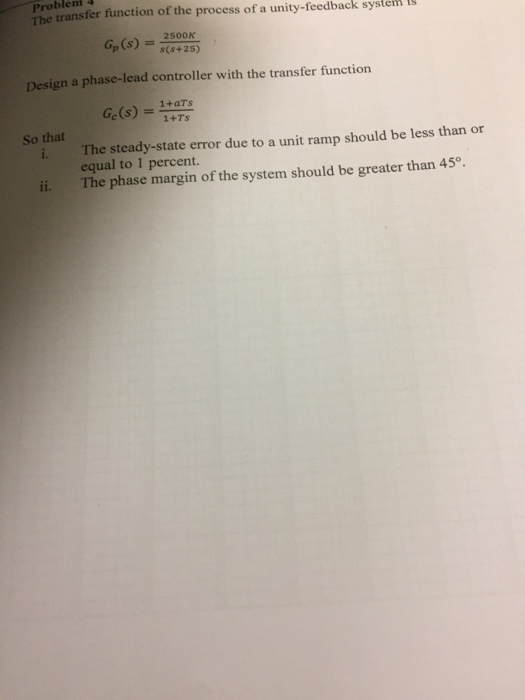Probleni 4 The transfer function of the process of a unity-feedback system 1 G, (s) = 2500K s(s+25) Design a phase-lead controller with the transfer function Ge (s) 1+aTs 1+Ts So that i. The steady-state error due to a unit ramp should be less than or ii. The phase margin of the system should be greater than 45 equal to 1 percent.

• ### Consider the electro-mechanical feedback control system shown in Figure 3. The voltage Ea(s) - Liea(t)) is...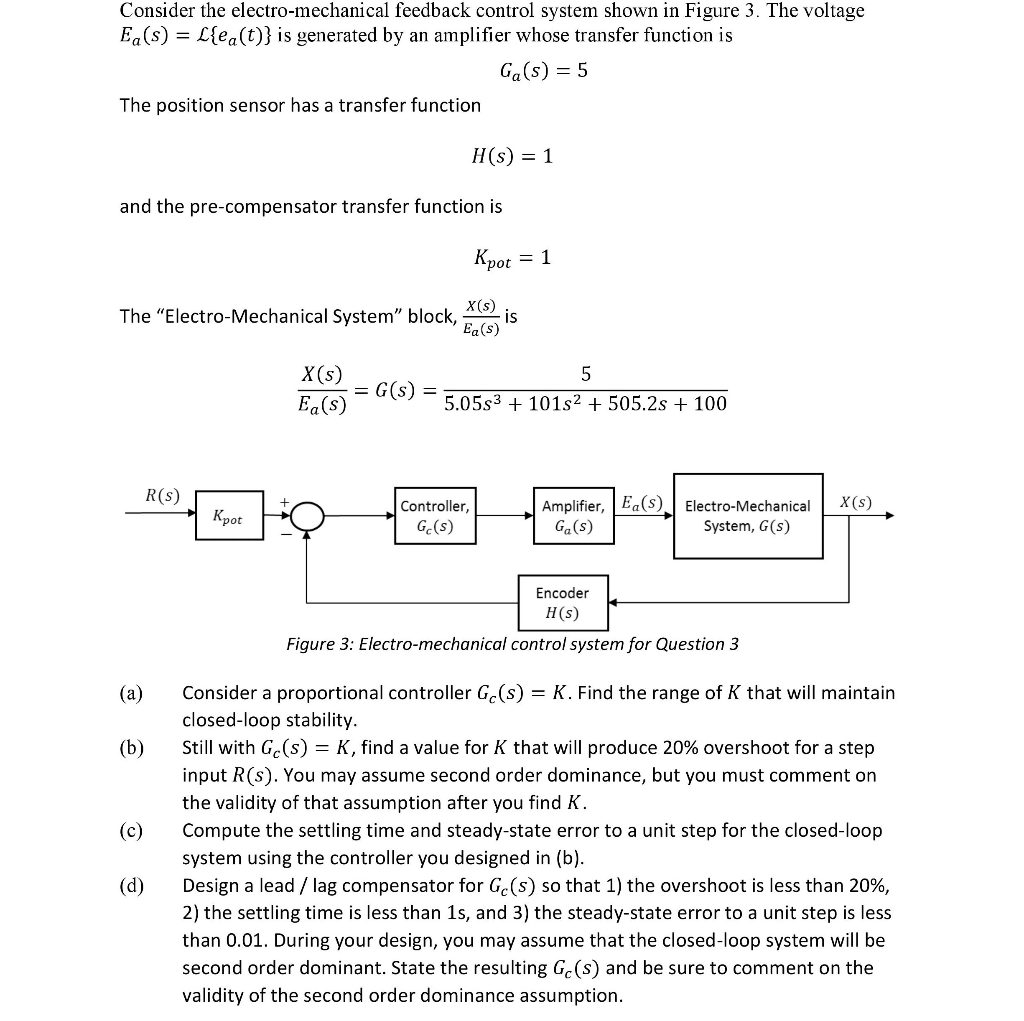Consider the electro-mechanical feedback control system shown in Figure 3. The voltage Ea(s) - Liea(t)) is generated by an amplifier whose transfer function is Ga(s) -5 The position sensor has a transfer function H(s) 1 and the pre-compensator transfer function is pot X (s) Ea(s) The "Electro-Mechanical System" block, is X(s) Ea(s) 5.05s3 101s2 +505.2s 100 R(s) Amplifier, |Ea(S)Electro-MechanicalX(S) Controller, Gc(s) K, pot Ga(s) System, G(s) Encoder H(s) Figure 3: Electro-mechanical control system for Question 3 Consider a proportional controller...

• ### 1. Consider a unity feedback control system with the transfer function G(s) = 1/[s(s+ 2)] in...

1. Consider a unity feedback control system with the transfer function G(s) = 1/[s(s+ 2)] in the forward path. (a) Design a proportional controller that yields a stable system with percent overshoot less that 5% for the step input (b) Find settling time and peak time of the closed-loop system designed in part (a); (c) Design a PD compensator that reduces the settling time computed in (b) by a factor of 4 while keeping the percent overshoot less that 5%...